Welcome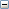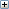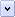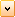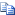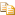ICreatePsplineSurfaceDLL Method (IBody2)

Creates a B-surface from a piecewise surface.

.NET Syntax

Visual Basic (Declaration)
Function ICreatePsplineSurfaceDLL( _
ByVal Dim As Integer, _
ByVal Uorder As Integer, _
ByVal VOrder As Integer, _
ByVal Ncol As Integer, _
ByVal Nrow As Integer, _
ByRef Coeffs As Double, _
ByVal Basis As Integer, _
ByRef Xform As Double, _
ByVal ScaleFactor As Double _
) As Surface
Visual Basic (Usage)
Dim instance As IBody2
Dim Dim As Integer
Dim Uorder As Integer
Dim VOrder As Integer
Dim Ncol As Integer
Dim Nrow As Integer
Dim Coeffs As Double
Dim Basis As Integer
Dim Xform As Double
Dim ScaleFactor As Double
Dim value As Surface

value = instance.ICreatePsplineSurfaceDLL(Dim, Uorder, VOrder, Ncol, Nrow, Coeffs, Basis, Xform, ScaleFactor)
C#
Surface ICreatePsplineSurfaceDLL(
int Dim,
int Uorder,
int VOrder,
int Ncol,
int Nrow,
ref double Coeffs,
int Basis,
ref double Xform,
double ScaleFactor
)
C++/CLI
Surface^ ICreatePsplineSurfaceDLL(
&   int Dim,
&   int Uorder,
&   int VOrder,
&   int Ncol,
&   int Nrow,
&   double% Coeffs,
&   int Basis,
&   double% Xform,
&   double ScaleFactor
)

Parameters

Dim

Dimension of the surface:

• 3 = nonrational
• 4 = rational
Uorder
Order in U
VOrder
Order in V
Ncol
Number of patches in U
Nrow
Number of patches in V
Coeffs
Coefficient array in the order that the Parasolid Kernel Interface routine  CRPWPS
needs them
Basis
Representation method; current default is polynomial
Xform
Transform to apply to the new surface
ScaleFactor
Units-of-measure scaling

Return Value

Newly created B-surface

Remarks

This method creates a B-surface from piecewise data.

As per Parasolid, The following ways to represent the data are available:

• Hermite (cubic only)  (basis = 13651)
• Bezier  (basis = 13652)
• Polynomial  (basis = 13653)
• Taylor series  (basis = 13654)

Dimension of coefficient vectors dim:

• For rational surfaces Dim = 4
• For non-rational surfaces Dim = 3

Order of each patch of the surface in u, Uorder, and in v, Vorder:

The order of the surface = degree + 1.

• The minimum order is 2.
• If the Hermite basis is used (basis = 13651) then the surface has to be bicubic (Uorder
= Vorder = 4).

Number of columns Ncol: There must be at least one column.

Number of rows Nrow: There must be at least one row.

Coefficient data Coeffs:

• Contains (Uorder * Vorder * Ncol * Nrow) vectors of dimension Dim. If Dim = 3, then the vectors are 3-D vectors giving the x, y and z components. If Dim = 4, then each vector has a weight (w) associated with it, and x, y, z and w components are supplied for each vector. The weights supplied must be greater than 0.
• The data is supplied patch by patch, row by row.
• The interpretation of the patch data depends on the representation method chosen; this is determined by the value of the argument basis.
• Adjacent patches (in the u and v directions) must meet all along the corresponding boundary; that is, the surface must be continuous.

Representation method basis:

The expressions for each patch of the B-surface P(u,v) in the various representations are shown next. For generality, the rational form is given. The simplification to the non-rational form can be obtained by setting both the weights and the denominator equal to 1.0.

• Bezier vertices 13652:

The equation of a rational Bezier surface patch is:

nu  nv                               nu nv

--  --                               / -- --

P(u,v)   =   >   >   b (u) b (v) w  V    /  >  >  b (u) b (v) w

--  --   i     j     ij ij           /   -- --  i    j     ij

i=0 j=0                              i=0 j=0

where nu  = uorder-1, nv = vorder-1

V   = Bezier vertex

ij

w   = weight for V

ij               ij

b (u), b (v) = Bezier coefficients.

i      j

For the rational form the Bezier vertices and weights are supplied:

V  ,w  ,V  ,w  ,...,V  ,w  ,V  ,w  ,...,V  ,w  ,...,V  ,w  ,...,V  ,w  .

00  00  10  10      m0  m0  01  01      m1  m1      0n  0n      mn  mn

For the non-rational form the w's are missed out.

• Polynomial coefficients 13653:

The surface equation is given by a rational bipolynomial of orders Uorder, Vorder:

nu  nv                          nu nv

--  --           i   j            / -- --      i  j

P(u,v)   =   >   >   w   A   u   v  /  >  >  w   u  v

--  --   ij  ij                  /   -- --  ij

i=0 j=0                           i=0 j=0

where nu = Uorder-1, nv = Vorder-1

For the rational form the polynomial coefficients Aij are supplied:

A  ,w  ,A  ,w  ,...,A  ,w  ,A  ,w  ,...,A  ,w  ,...,A  ,w  ,...,A  ,w

00  00  10  10      m0  m0  01  01      m1  m1      0n  0n      mn  mn

starting with the constant term and ending with the term of highest degree.

For the non-rational form the w's are missed out.

• Hermite coefficients 13651

This method can only be used for bicubics.

The Hermite equation for the patch in matrix form is:

2  3       T            2 3  T

( 1 u u  u ) M A M ( 1 v v v )

P(u,v) = -------------------------------------

2  3       T       2 3  T

( 1 u u  u ) M W M ( 1 v v v )

where M =  (  1  0  0  0  )

(  0  0  1  0  )

( -3  3 -2 -1  )

(  2 -2  1  1  )

A =  ( w00*P00   w01*P01   wv00*Pv00   wv01*Pv01  )

( w10*P10   w11*P11   wv10*Pv10   wv11*Pv11  )

( wu00*Pu00 wu01*Pu01 wuv00*Puv00 wuv01*Puv01 )

( wu10*Pu10 wu11*Pu11 wuv10*Puv10 wuv11*Puv11 )

W = ( w00  w01  wv00  wv01 )

( w10  w11  wv10  wv11 )

( wu00 wu01 wuv00 wuv01 )

( wu10 wu11 wuv10 wuv11 )

and the superscript T denotes the transpose.

In the matrices A and W, the coefficients P, Pu, Pv and Puv are the points at the corners and their derivatives. The w's are the corresponding weights and their derivatives. P00 denotes P(0,0), and so on.

For the rational form the coefficients are supplied:

P00,   w00,   P10,   w10,   P01,   w01,   P11,   w11

Pu00,  wu00,  Pu10,  wu10,  Pu01,  wu01,  Pu11,  wu11

Pv00,  wv00,  Pv10,  wv10,  Pv01,  wv01,  Pv11,  wv11

Puv00, wuv00, Puv10, wuv10, Puv01, wuv01, Puv11, wuw11

For the non-rational form, the w's are missed out.

• Taylor series 13654:

This method stores the derivatives evaluated u=0, v=0 corner of each patch,  allowing the surface to be reconstructed as a Taylor series:

nu    nv

--     --    (i)(j)  (i)(j)  i  j       /

>      >    w       P       u  v   /  i! j!

--     --

i=0   j=0

P(u,v) = ------------------------------------------

nu    nv

--    --     (i)(j)  i  j       /

>     >     w       u  v   /  i! j!

--    --

i=0  j=0

where nu = Uorder-1, nv = Vorder-1

i+j

(i)(j)     d   P

P         =  ------ (0,0)

i  j

du dv

i+j

(i)(j)     d   w

w         = ------ (0,0)

i  j

du dv

The point is supplied first, followed by the U derivatives in order and ending with the derivative of order Uorder-1 in U, Vorder-1 in V.

Any existing object created by this method is destroyed if you call this method again.

Availability

SolidWorks 2001Plus FCS, Revision Number 10.0

Provide feedback on this topic

SOLIDWORKS welcomes your feedback concerning the presentation, accuracy, and thoroughness of the documentation. Use the form below to send your comments and suggestions about this topic directly to our documentation team. The documentation team cannot answer technical support questions. Click here for information about technical support.

* Required

 *Email: Subject: Feedback on Help Topics Page: ICreatePsplineSurfaceDLL Method (IBody2) *Comment: * I acknowledge I have read and I hereby accept the privacy policy under which my Personal Data will be used by Dassault Systèmes

Print Topic

Select the scope of content to print:

x

We have detected you are using a browser version older than Internet Explorer 7. For optimized display, we suggest upgrading your browser to Internet Explorer 7 or newer.

Never show this message again
x

Web Help Content Version: API Help (English only) 2010 SP05

To disable Web help from within SOLIDWORKS and use local help instead, click Help > Use SOLIDWORKS Web Help.

To report problems encountered with the Web help interface and search, contact your local support representative. To provide feedback on individual help topics, use the “Feedback on this topic” link on the individual topic page.